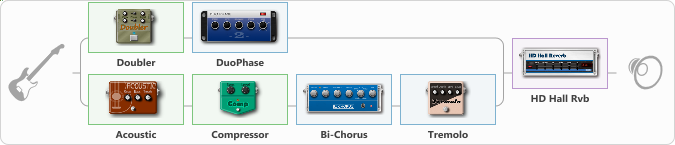# Heaven Eleven

Discussion in 'ToneLib-GFX presets' started by topotoo, Feb 9, 2020.

1. Heaven Eleven

Preset name: Heaven Eleven

Clean max reverb sound

Effects chain:Effect: "Splitter" (Dynamics / Filter), active - "yes"
{
"A-Bypass" = Off
"A-Pan" = 0
"A-Level" = 55
"B-Bypass" = Off
"B-Pan" = 0
"B-Level" = 55

Left branch:
{

Effect: "Doubler" (Dynamics / Filter), active - "yes"
{
"A-Dly" = 75
"A-Inv" = Off
"A-Level" = 75
"B-Dly" = 50
"B-Inv" = Off
"B-Level" = 50
}

Effect: "DuoPhase" (Modulation / Sfx), active - "yes"
{
"Speed A" = 4.1
"Speed B" = 2.1
"Depth" = 76
"Reso" = 15
"Mode" = Parallel
}
}
Right branch:
{

Effect: "Acoustic" (Dynamics / Filter), active - "yes"
{
"Style" = Arpeggio/Classic
"Resonance" = 67
"Bass" = 19
"Treble" = 55
}

Effect: "Compressor" (Dynamics / Filter), active - "yes"
{
"Sense" = 16
"Level" = 50
}

Effect: "Bi-Chorus" (Modulation / Sfx), active - "yes"
{
"Speed A" = 3.2
"Speed B" = 4.0
"Depth" = 43
"Reso" = 0
"Mode" = Parallel
"Mix" = 56
}

Effect: "Tremolo" (Modulation / Sfx), active - "yes"
{
"Speed" = 5.9
"Depth" = 50
"Level" = 68
}
}
}

Effect: "HD Hall Rvb" (Reverb), active - "yes"
{
"Time" = 8.2
"PreLPF" = 69
"PreDelay" = 0
"HiDamp" = 0
"LoGain" = 1.9
"Mix" = 91
}

Note: You will need to download and install the ToneLib-GFX software to use the preset.

#### Attached Files:

• ###### Heaven_Eleven.tlgfx
File size:
804 bytes
Views:
13,325
CosmycKit, Gizmoli, Romka999 and 77 others like this.